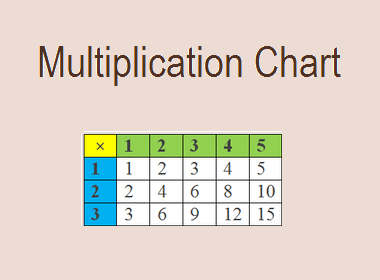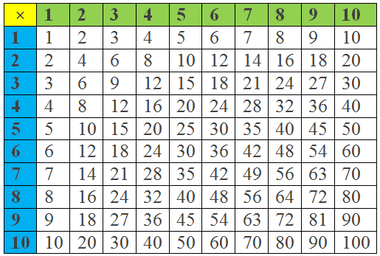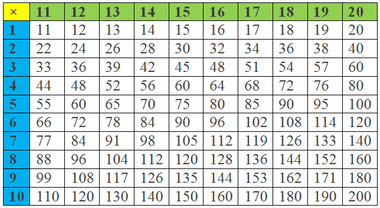# Multiplication ChartA multiplication chart, also known as a times table or multiplication table, is a visual tool that displays the products of numbers from 1 to a certain range, typically up to 10 or 12. It is organized in a grid format with the numbers listed along the top and left sides. The chart shows the results of multiplying the numbers in each row with the numbers in each column, resulting in a grid of multiplication facts. Each cell in the chart represents the product of the corresponding row and column numbers.

For example, in a multiplication chart that goes up to 10, the number 3 in the first row and the number 4 in the first column intersect at the cell containing the number 12. This indicates that 3 multiplied by 4 equals 12. Similarly, the chart provides the products for all possible combinations of numbers within the given range.

********************

********************

A multiplication chart is a helpful tool for students learning multiplication as it provides a clear and organized representation of the multiplication facts. It allows students to quickly find the product of two numbers by locating the corresponding cell in the chart. The chart also reveals patterns and relationships between numbers, aiding in the understanding of multiplication concepts and facilitating the memorization of multiplication facts.

By regularly referring to the multiplication chart, students can reinforce their knowledge of multiplication, improve fact fluency, and develop a strong foundation for more complex mathematical operations.

Multiplication Chart 1 - 10Multiplication Chart 11 – 20Benefits of the Multiplication Chart:

1.    Visual Representation: The chart offers a visual representation of multiplication, allowing students to see the relationship between numbers and their products. This visual aid enhances comprehension and makes abstract concepts more tangible.

2.    Pattern Recognition: The multiplication chart reveals numerous patterns and symmetries. For instance, multiples of the same number form diagonal lines across the chart. Recognizing these patterns helps students identify relationships and develop mental shortcuts for solving multiplication problems efficiently.

3.    Memorization Aid: The chart serves as a valuable tool for memorizing multiplication facts. By regularly referring to the chart, students reinforce their knowledge of multiplication, gradually reducing their reliance on it as they internalize the facts.

4.    Multiplication Strategies: The chart supports the development of multiplication strategies, such as skip counting, grouping, and repeated addition. These strategies enable students to approach multiplication problems from different angles, fostering a deeper understanding of the concept.

Applications in Education:

1.    Introduction to Multiplication: The multiplication chart is often introduced to students at an early stage of their mathematical journey. By exploring the chart, young learners grasp the concept of multiplication, observe number patterns, and develop a solid foundation for future mathematical concepts.

2.    Fact Fluency: Mastering multiplication facts is essential for more complex mathematical operations. The chart helps students build fluency by providing a visual reference and promoting regular practice.

3.    Mental Math Skills: The multiplication chart aids in mental math development. By internalizing the chart's patterns, students can quickly recall multiplication facts and perform mental calculations with ease.

4.    Problem-Solving: The chart expands students' problem-solving capabilities. By identifying patterns and making connections, learners gain the ability to apply multiplication strategies to real-world scenarios and mathematical puzzles.

5.    Multiplicative Reasoning: Through the exploration of the chart, students develop multiplicative reasoning skills, enabling them to understand the relationship between multiplication, division, factors, and multiples.

Teaching Strategies:

Educators can employ various strategies to maximize the benefits of the multiplication chart:

1.    Interactive Exploration: Encourage students to actively engage with the multiplication chart by exploring the patterns and discussing their observations. This interactive approach fosters critical thinking and mathematical discourse.

2.    Hands-On Activities: Incorporate hands-on activities that involve manipulating the chart, such as highlighting specific number patterns or filling in missing values. These activities promote a deeper understanding of multiplication concepts.

3.    Game-Based Learning: Utilize educational games and puzzles that incorporate the multiplication chart. These interactive and engaging activities make learning multiplication enjoyable while reinforcing conceptual understanding.

4.    Gradual Fade: As students become more proficient in multiplication, gradually reduce their reliance on the chart. Encourage mental calculations and challenge them to recall multiplication facts from memory.

Conclusion:

A multiplication chart is a powerful tool that facilitates the understanding, mastery, and application of multiplication. Its visual representation, pattern recognition, and support for mental math skills make it an invaluable asset in mathematics education. By leveraging the benefits of the multiplication chart, educators can empower students to develop a strong foundation in multiplication, laying the groundwork for success in higher-level mathematics and problem-solving.